Levels »

# Beware of Low-Flying Nightmares

### The Catacombs, Elevation: -7.5m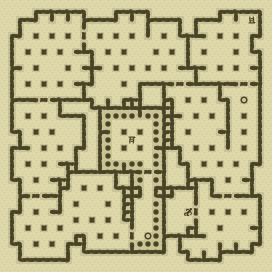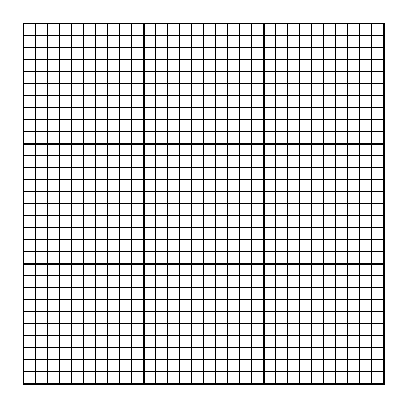### Items

•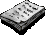Nazi Propaganda (22, 25) (on Anonymous German)
•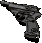Rusted Walther P4 (22, 25) (on Anonymous German)
•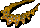Diamond Necklace (13, 9)
•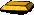11 Gold Ingots (21, 23) - (21, 23) - (20, 24) - (20, 24) - (21, 25) - (20, 26) - (21, 26) - (22, 26) - (20, 27) - (21, 27) - (21, 27)
•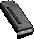Walther P4 Magazine (3 rounds) (22, 25) x3 (in Rusted Walther P4) (on Anonymous German)
•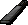MP-41 Magazine (32 rounds) (14, 1) x32

•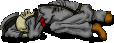(22, 25) Anonymous German
•Rusted Walther P4
•Walther P4 Magazine x3
•Nazi Propaganda

### Monsters

•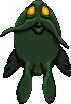26 Nightmares (Random rate: 3) (7, 2) - (8, 2) - (8, 3) - (4, 4) - (8, 4) - (20, 10) - (21, 10) - (22, 10) - (23, 11) - (21, 12) - (22, 14) - (25, 14) - (2, 21) - (4, 21) - (3, 22) - (5, 22) - (3, 24) - (4, 24) - (2, 25) - (4, 25) - (1, 26) - (3, 27) - (1, 28) - (2, 30) - (3, 30) - (4, 30)
•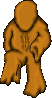24 Oozes (Random rate: 3) (8, 1) - (29, 1) - (20, 2) - (29, 2) - (30, 2) - (12, 3) - (23, 4) - (1, 6) - (14, 8) - (2, 12) - (26, 12) - (30, 13) - (3, 16) - (22, 17) - (28, 18) - (2, 22) - (4, 22) - (30, 28) - (2, 29) - (22, 29) - (27, 29) - (29, 29) - (23, 30) - (25, 30)
•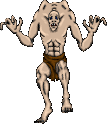Ghouls (Random rate: 5)

### Locations

• 15 Door openers (8, 3) - (10, 3) - (21, 8) - (29, 8) - (4, 10) - (21, 10) - (29, 10) - (4, 12) - (17, 19) - (17, 21) - (3, 22) - (27, 22) - (3, 24) - (14, 28) - (16, 28)
• Gold key-operated door opener (24, 25)
• 2 Save runes(29, 11) - (17, 28)